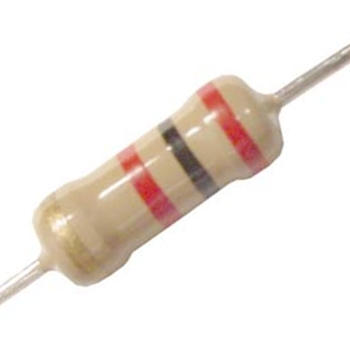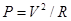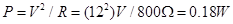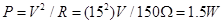﻿ What is the Power Rating of a Resistor?# What is the Power Rating of a Resistor?The power rating of a resistor is the specification given with a resistor that serves to tell the maximum amount of power that the resistor can withstand. Thus, if a resistor has a power rating of 1/4 watts, 1/4 watts is the maximum amount of power that should be fed into the resistor.

When current passes through electrical components, it normally generates heat. If the current is small enough and suitable for the circuit, this heat is usually negligible and unnoticed in a circuit. But if the current is large enough, it can create a substantial amount of heat in a circuit. The current can melt components and possibly create shorts in a circuit. This is why resistors are given power ratings— to specify the maximum allowable amount of power that can pass through it. If this wattage of power is exceeded, the resistor may not be able to withstand the power and may melt and can create a short in a circuit, which can lead to even greater hazards for the circuit.

Let's now define power so that we know exactly what is meant when power is talked about. Power is defined as the electrical energy that a circuit is capable of delivering. The equation that shows the power of a circuit is P=VI where P is power, V is voltage, and I is current. Alternatively, since ohm's law can be substituted into the this equation, power is also expressed asand. We can use these formulas to determine what power a circuit will be operating at and thus we can know what power rating we need for a resistor.

Let's look at some examples right now of resistors and the power ratings we would need for them for you to get a practical idea:
-Let's say we have a 800Ω resistor with a voltage of 12 volts powering the circuit to light an LED. Neglecting the wire and LED resistance, which are negligible, the power that the circuit would be delivering is:Here, a 1/4 watt resistor would suffice and be suitable for the circuit.
-Let's say now we have a 150Ω resistor with 15 volts powering the circuit to control a motor. The power that the circuit would be delivering to the motor is:Here, a 2 watt resistor would be suitable for the circuit. A lower power rated resistor such as a 0.25W, 0.5W, or 1W resistor would most likely cause the circuit to smoke, since the resistor would receive more power than it could handle.

Usually in electronic circuits, the power rating is not considered because the standard 0.25W resistor is normally suitable, since electronic circuits, for the vast majority, deal with low voltage and low current; and thus low power. But in cases of circuits with high voltage and low resistance (high power), power ratings of resistors should be carefully chosen since more power is delivered in the circuit. Always choose a resistor with a higher power rating than the power being used in the circuit so that the resistor isn't destroyed by excess heat; this would only serve to cause other hazards or malfunctions in the circuit.

The common standard power ratings of resistors are 0.25W, 0.5W, 1W, 2W, 5W, and 25W. So the circuit designer must choose accordingly for the circuit.

Related Resources